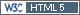## Function CVpooled (package PowerTOST) [R for BE/BA]

Dear all! I am new to R and RStudio. It will be very useful to me if you help me with the following questions:

1. Here is an example of my data:

``` CVs <- ("  PKmetric | CV     | n  |design|source        Cmax  | 0.2617 | 23 | 2x2  | study 1     Cmax  | 0.1216 | 24 | 2x2  | study 2     Cmax  | 0.1426 | 24 | 2x2  | study 3     Cmax  | 0.1480 | 27 | 3x3  | study 4     Cmax  | 0.1476 | 27 | 3x3  | study 4a      Cmax  | 0.2114 | 18 | 2x2  | study 5 ") txtcon <- textConnection(CVs) CVdata <- read.table(txtcon, header=TRUE, sep="|", strip.white=TRUE, as.is=TRUE) close(txtcon) CVsCmax <- subset(CVdata, PKmetric=="Cmax") CVpooled(CVsCmax, alpha=0.2, logscale=TRUE) print(CVpooled(CVsCmax, alpha=0.2, robust=TRUE), digits=6, verbose=TRUE)```

The result I got:

```CVpooled(CVsCmax, alpha=0.2, logscale=TRUE) 0.1677 with 181 degrees of freedom   print(CVpooled(CVsCmax, alpha=0.2, robust=TRUE), digits=6, verbose=TRUE) Pooled CV = 0.175054 with 129 degrees of freedom (robust df's) Upper 80% confidence limit of CV = 0.185309```

Why are degrees of freedom so huge? And why did I get two different results 0.1677 and 0.1750? Is it acceptable and can I use any result to calculate sample size in PowerTOST? What result must I choose (0.1677, 0.1750, 0.1853) for calculating sample size?

2. And the second question. Is argument "digits" from function CVpooled simply a number of observations for a variable?

Thank you in advance!Ing. Helmut Schütz